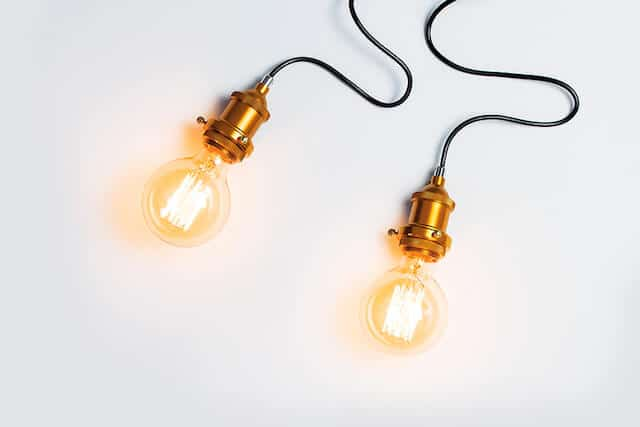Can you imagine the world without electricity? In our modern society, electricity is something we use every day. Electrical appliances at home transfer energy from the main supply to heat or light our homes and streets. We need it to power our devices like lamps and televisions, or transport and healthcare equipment.

Electrical energy and electrical power are two major terms associated with practical electricity. So what is the difference and how do they work? Read on to find out!

Electrical power

Electrical power, P, is defined as the rate of work done, W, in an electrical system over time, t. It is measured in Watts (W), or sometimes also described as joules per second (J/s). This relationship can be described by the formula below:

• Formula: Power (W) = Work done (J) / Time (s)
• Simplified formula: P = W/t

We can also use principles of Ohm’s Law to derive the formula for electrical power:

• Formula: Power (W) = Voltage (V) x Current (A)
• Simplified formula: P = VI
• Derived formulae: P = VI = I2R = V2/R

Electrical energy

In an electrical circuit, electrical energy is defined as the overall work done. The SI unit of electrical energy is Joules (J). It is the product of power multiplied by the length of time it was consumed. Therefore the formula is:

• Formula: Energy (J) = Power (W) x Time (s)
• Simplified formula: E = Pt
• Derived formulae: E = Pt = VIt = I2Rt= V2t/R

Heating effect of electricity

Electrical energy can be converted into other forms of energy by using different electrical appliances. When the electrical current passes through a conductor, heat energy is generated, this is called the heating effect. Some examples of applications of the heating effect in our homes are electric kettles, electric irons, and water heaters.

Unsafe use of electricity can cause hazards like fires and shocks, which could be dangerous. This can happen when there is a short circuit.

Parts inside a plug

The plug of the electricity supplies in our homes have three wires – Live, Neutral, and Earth wires, and a fuse. The wires are brown, blue, and green in colour, respectively. The purpose of these wires are:

• Live wire: To carry the current from the electricity supply to the appliance. In household items, switches are fitted onto the live wire so that opening it will disconnect the appliance from the high voltages. This prevents the user from getting electrocuted if he accidently touches it. A fuse should be placed in the live wire too.
• Neutral wire: To complete the circuit by carrying current from the appliance back to the generator.
• Earth wire: To prevent people from getting electrocuted by providing an alternative path for current to flow when there is a current leakage. It does not carry any electricity.

The purpose of the fuse is to protect appliances from damage caused by large electric currents. Inside the fuse, there is a short wire that will melt if the current exceeds its fuse rating. Both the earth wire and fuse are safety features to protect the users from any hazards.

Conclusion

The concepts of practical electricity are interesting as you will start to notice that they are applied everywhere. Now, when you look at your table lamp or iron your clothes, you have the basic knowledge of how they work. A physics tuition teacher will be able to help you understand the concepts deeper and answer the questions you have. If your exams are coming, O level physics tuition can help you learn in a smart and fun way!